Related Articles

# Finding inverse of a matrix using Gauss – Jordan Method | Set 2

• Last Updated : 02 Nov, 2020

Given a Matrix, the task is to find the inverse of this Matrix using the Gauss-Jordan method.
What is matrix?

Matrix is an ordered rectangular array of numbers.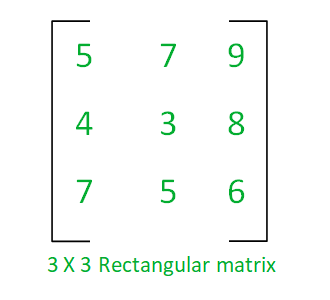Operations that can be performed on a matrix are: Addition, Subtraction, Multiplication or Transpose of matrix etc.

### Inverse of a matrix:

Given a square matrix A, which is non-singular (means the Determinant of A is nonzero); Then there exists a matrixwhich is called inverse of matrix A.
The inverse of a matrix is only possible when such properties hold:

1. The matrix must be a square matrix.
2. The matrix must be a non-singular matrix and,
3. There exist an Identity matrix I for whichIn general, the inverse of n X n matrix A can be found using this simple formula: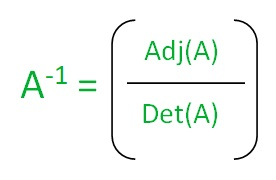where, Adj(A) denotes the adjoint of a matrix and, Det(A) is Determinant of matrix A.

### Methods for finding Inverse of Matrix:

Finding the inverse of a 2×2 matrix is a simple task, but for finding the inverse of larger matrix (like 3×3, 4×4, etc) is a tough task, So the following methods can be used:

1. Elementary Row Operation (Gauss-Jordan Method) (Efficient)
2. Minors, Cofactors and Ad-jugate Method (Inefficient)

### Elementary Row Operation (Gauss – Jordan Method):

Gauss-Jordan Method is a variant of Gaussian elimination in which row reduction operation is performed to find the inverse of a matrix.
Steps to find the inverse of a matrix using Gauss-Jordan method:
In order to find the inverse of the matrix following steps need to be followed:

1. Form the augmented matrix by the identity matrix.
2. Perform the row reduction operation on this augmented matrix to generate a row reduced echelon form of the matrix.
3. The following row operations are performed on augmented matrix when required:
• Interchange any two row.
• Multiply each element of row by a non-zero integer.
• Replace a row by the sum of itself and a constant multiple of another row of the matrix.

Example:

•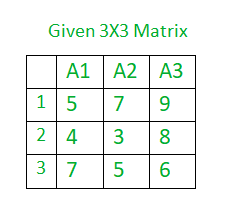• Augmented Matrix is formed as A:B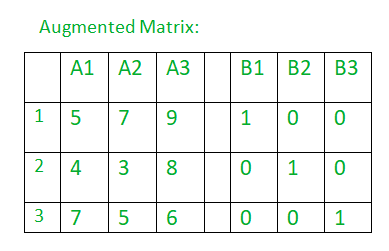• After applying the Gauss-Jordan elimination method: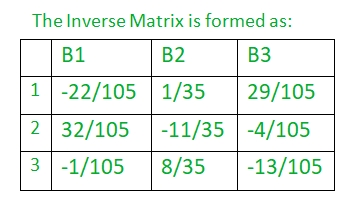Below is the C++ program to find the inverse of a matrix using the Gauss-Jordan method:

## CPP

 `// C++ program to find the inverse of Matrix.` `#include ``#include ``using` `namespace` `std;` `// Function to Print matrix.``void` `PrintMatrix(``float``** ar, ``int` `n, ``int` `m)``{``    ``for` `(``int` `i = 0; i < n; i++) {``        ``for` `(``int` `j = 0; j < m; j++) {``            ``cout << ar[i][j] << ``"  "``;``        ``}``        ``printf``(``"\n"``);``    ``}``    ``return``;``}` `// Function to Print inverse matrix``void` `PrintInverse(``float``** ar, ``int` `n, ``int` `m)``{``    ``for` `(``int` `i = 0; i < n; i++) {``        ``for` `(``int` `j = n; j < m; j++) {``            ``printf``(``"%.3f  "``, ar[i][j]);``        ``}``        ``printf``(``"\n"``);``    ``}``    ``return``;``}` `// Function to perform the inverse operation on the matrix.``void` `InverseOfMatrix(``float``** matrix, ``int` `order)``{``    ``// Matrix Declaration.` `    ``float` `temp;` `    ``// PrintMatrix function to print the element``    ``// of the matrix.``    ``printf``(``"=== Matrix ===\n"``);``    ``PrintMatrix(matrix, order, order);` `    ``// Create the augmented matrix``    ``// Add the identity matrix``    ``// of order at the end of original matrix.``    ``for` `(``int` `i = 0; i < order; i++) {` `        ``for` `(``int` `j = 0; j < 2 * order; j++) {` `            ``// Add '1' at the diagonal places of``            ``// the matrix to create a identity matirx``            ``if` `(j == (i + order))``                ``matrix[i][j] = 1;``        ``}``    ``}` `    ``// Interchange the row of matrix,``    ``// interchanging of row will start from the last row``    ``for` `(``int` `i = order - 1; i > 0; i--) {` `        ``// Swapping each and every element of the two rows``        ``// if (matrix[i - 1] < matrix[i])``        ``// for (int j = 0; j < 2 * order; j++) {``        ``//``        ``//        // Swapping of the row, if above``        ``//        // condition satisfied.``        ``// temp = matrix[i][j];``        ``// matrix[i][j] = matrix[i - 1][j];``        ``// matrix[i - 1][j] = temp;``        ``//    }` `        ``// Directly swapping the rows using pointers saves``        ``// time` `        ``if` `(matrix[i - 1] < matrix[i]) {``            ``float``* temp = matrix[i];``            ``matrix[i] = matrix[i - 1];``            ``matrix[i - 1] = temp;``        ``}``    ``}` `    ``// Print matrix after interchange operations.``    ``printf``(``"\n=== Augmented Matrix ===\n"``);``    ``PrintMatrix(matrix, order, order * 2);` `    ``// Replace a row by sum of itself and a``    ``// constant multiple of another row of the matrix``    ``for` `(``int` `i = 0; i < order; i++) {` `        ``for` `(``int` `j = 0; j < order; j++) {` `            ``if` `(j != i) {` `                ``temp = matrix[j][i] / matrix[i][i];``                ``for` `(``int` `k = 0; k < 2 * order; k++) {` `                    ``matrix[j][k] -= matrix[i][k] * temp;``                ``}``            ``}``        ``}``    ``}` `    ``// Multiply each row by a nonzero integer.``    ``// Divide row element by the diagonal element``    ``for` `(``int` `i = 0; i < order; i++) {` `        ``temp = matrix[i][i];``        ``for` `(``int` `j = 0; j < 2 * order; j++) {` `            ``matrix[i][j] = matrix[i][j] / temp;``        ``}``    ``}` `    ``// print the resultant Inverse matrix.``    ``printf``(``"\n=== Inverse Matrix ===\n"``);``    ``PrintInverse(matrix, order, 2 * order);` `    ``return``;``}` `// Driver code``int` `main()``{``    ``int` `order;` `    ``// Order of the matrix``    ``// The matrix must be a square a matrix``    ``order = 3;``    ``/*``float matrix = { { 5, 7, 9 },``                         ``{ 4, 3, 8 },``                         ``{ 7, 5, 6 },``                         ``{ 0 } };``*/``    ``float``** matrix = ``new` `float``*;``    ``for` `(``int` `i = 0; i < 20; i++)``        ``matrix[i] = ``new` `float``;` `    ``matrix = 5;``    ``matrix = 7;``    ``matrix = 9;``    ``matrix = 4;``    ``matrix = 3;``    ``matrix = 8;``    ``matrix = 7;``    ``matrix = 5;``    ``matrix = 6;` `    ``// Get the inverse of matrix``    ``InverseOfMatrix(matrix, order);` `    ``return` `0;``}`
Output:
```=== Matrix ===
5  7  9
4  3  8
7  5  6

=== Augmented Matrix ===
7  5  6  0  0  1
5  7  9  1  0  0
4  3  8  0  1  0

=== Inverse Matrix ===
-0.210  0.029  0.276
0.305  -0.314  -0.038
-0.010  0.229  -0.124

```

Attention reader! Don’t stop learning now. Get hold of all the important DSA concepts with the DSA Self Paced Course at a student-friendly price and become industry ready.  To complete your preparation from learning a language to DS Algo and many more,  please refer Complete Interview Preparation Course.

In case you wish to attend live classes with experts, please refer DSA Live Classes for Working Professionals and Competitive Programming Live for Students.

My Personal Notes arrow_drop_up